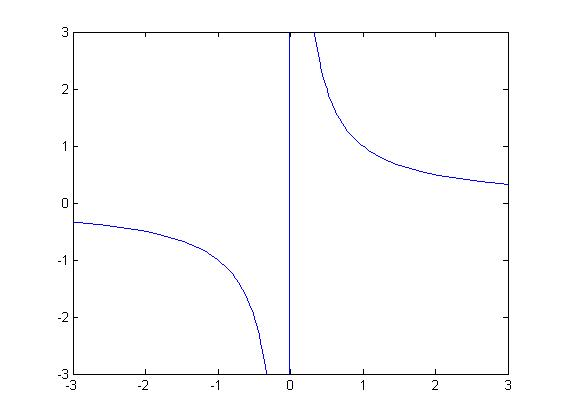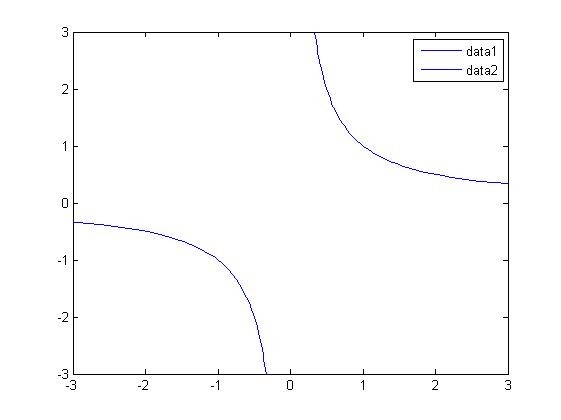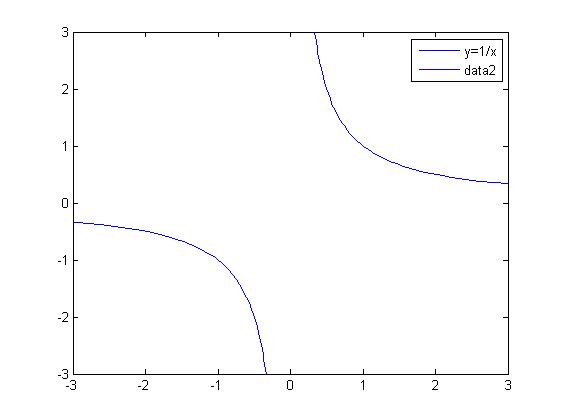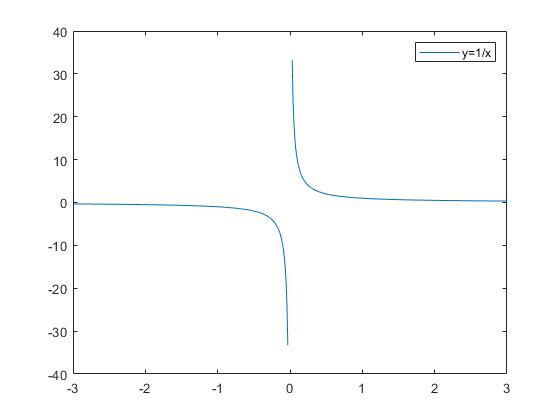2021-07-11 20:51

# matlab用syms绘制分段函数，用fplot绘制不在定义域的函数曲线fplot(f,[-3,3,-3,3])
fplot(@(x)1/x,[-3,0,-3,3]);hold on;
fplot(@(x)1/x,[-0,3,-3,3]);
axis([-3,3,-3,3])legend('y=1/x');• 写回答
• 好问题 提建议
• 追加酬金
• 关注问题
• 邀请回答

#### 2条回答默认 最新

•CSDN专家-Matlab_Fans 2021-07-11 21:18
最佳回答

解决办法：使用nan非数进行镂空

``````x = linspace(-3,3,500);
f = @(x) 1./x;
for ii = 1:length(x)
if  abs(x(ii))<0.03
x(ii) = nan;
y(ii) = nan;
end
end
plot(x,f(x))
legend('y=1/x')
``````评论
解决 无用
打赏 举报